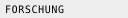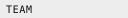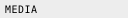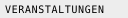Forschung

#### Robot localizationOne of the subtasks in robot soccer and current research topic is self-localization, i.e. to determine the position and orientation of the robot. In robot soccer the robots have to localize on the playing field using landmarks such as the goals, field lines, and the colored beacons. These landmarks are not necessarily unique. Determining position and orientation efficiently and accurately while having to rely on noisy landmark observations poses a difficult problem.

The so-called Monte-Carlo approach is a Markov-localization method which is commonly used for self-localization in robot soccer. It is a probabilistic approach, in which the current location of the robot is modeled as the density of a set of particles. Each particle can be seen as the hypothesis of the robot being located at this posture. Therefore, the particles consist of a robot pose, i. e. a vector representing the robot's x/y-coordinates and its rotation.

A Markov-localization method requires both an observation model and a motion model. The observation model describes the probability for taking certain measurements at certain locations. The motion model expresses the probability for certain actions to move the robot to certain relative postures.

The localization approach works as follows: first, all particles are moved according to the motion model of the previous action of the robot. Then, the probabilities for all particles are determined on the basis of the observation model for the current sensor readings, i. e. bearings on landmarks calculated from the actual camera image. Based on these probabilities, the so-called resampling is performed, i. e. moving more particles to the locations of particles with a high probability. Afterwards, the average of the probability distribution is determined, representing the best estimation of the current robot pose. Finally, the process repeats from the beginning.